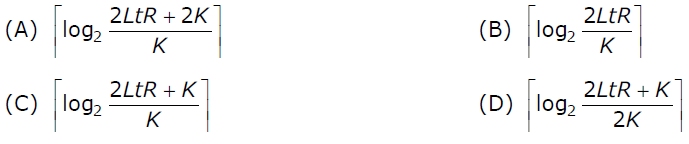# GATE | GATE-CS-2007 | Question 69

The distance between two stations M and N is L kilometers. All frames are K bits long. The propagation delay per kilometer is t seconds. Let R bits/second be the channel capacity. Assuming that processing delay is negligible, the minimum number of bits for the sequence number field in a frame for maximum utilization, when the sliding window protocolis used, is:(A) A
(B) B
(C) C
(D) D